Contemporary Mathematics

# 4.2Early Numeration Systems

Contemporary Mathematics4.2 Early Numeration Systems

Figure 4.3 Babylonians used clay tablets for writing and record keeping. (credit: modification of work by Osama Shukir Muhammed Amin FRCP(Glasg), CC BY 4.0 International)

### Learning Objectives

After completing this section, you should be able to:

1. Understand and convert Babylonian numerals to Hindu-Arabic numerals.
2. Understand and convert Mayan numerals to Hindu-Arabic numerals.
3. Understand and convert between Roman numerals and Hindu-Arabic numerals.

Each culture throughout history had to develop its own method of counting and recording quantity. The system used in Australia would necessarily differ from the system developed in Babylon that would, in turn, differ from the system developed in sub-Saharan Africa. These differences arose due to cultural differences. In nearly all societies, knowing the difference between one and two would be useful. But it might not be useful to know the difference between 145 and 167, as those quantities never had a practical use. For example, a shepherd likely didn't manage more than 100 sheep, so quantities larger than 100 might never have been encountered. This can even be seen in our use of the term few, which is an inexact quantity that most would agree means more than two. However, as societies became more complex, as commerce arose, as military bodies developed, so did the need for a system to handle large numbers. No matter the system, the issues of representing multiple values and how many symbols to use had to be addressed. In this section, we explore how the Babylonians, Mayans, and Romans addressed these issues.

### Understand and Convert Babylonian Numerals to Hindu-Arabic Numerals

The Babylonians used a mix of an additive system of numbers and a positional system of numbers. An additive system is a number system where the value of repeated instances of a symbol is added the number of times the symbol appears. A positional system is a system of numbers that multiplies a “digit” by a number raised to a power, based on the position of the “digit.”

The Babylonian place values didn’t use powers of 10, but instead powers of 60. They didn’t use 60 different symbols though. For the value 1, they used the following symbol: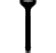For values up to 9, that symbol would be repeated, so three would be written asTo represent the quantity 10, they used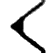For 20, 30, 40, and 50, they repeated the symbol for 10 however many times it was needed, so 40 would be writtenWhen they reached 60, they moved to the next place value. The complete list of the Babylonian numerals up to 59 is in Table 4.1.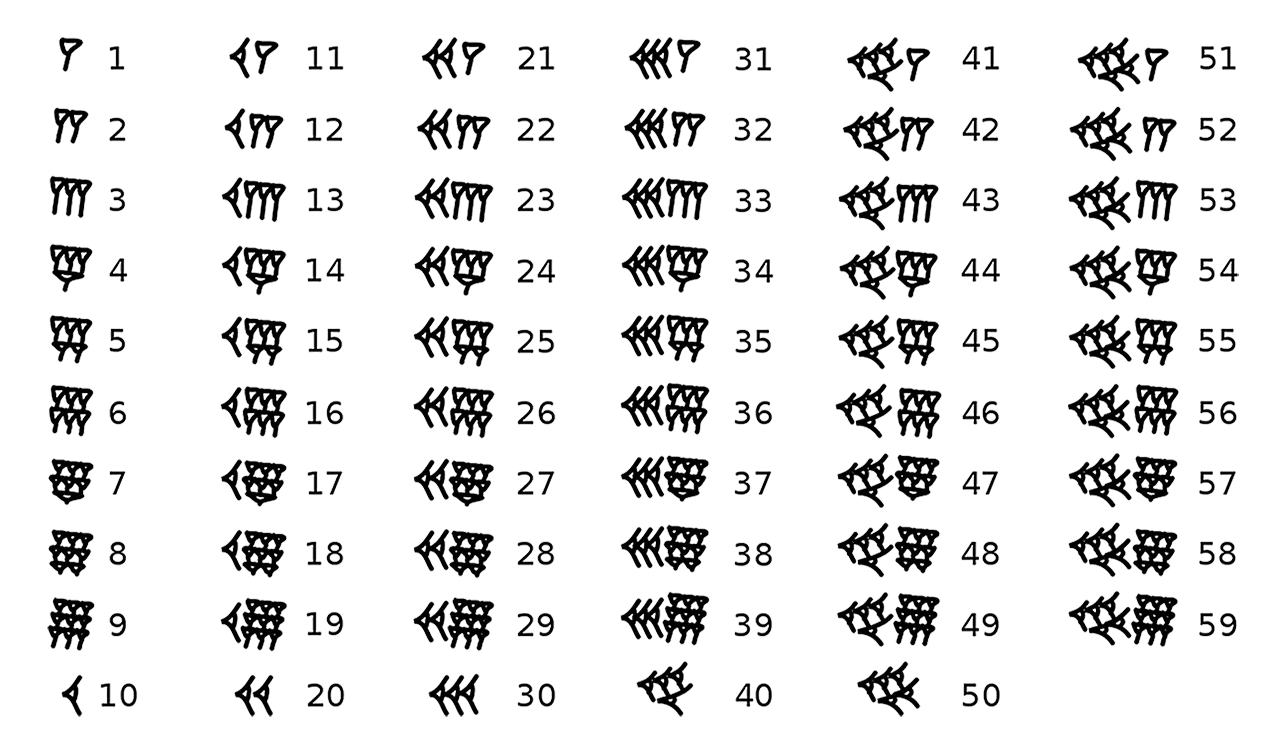Table 4.1 Babylonian Numerals

You can see how Babylonians repeated the symbols to indicate multiples of a value. The number 6 is 6 of the symbol for 1 grouped together. The symbol for 30 is three of the symbols for 10 grouped together. However, their system doesn’t go past 59. To go past 59, they used place values. As opposed to the Hindu-Arabic system, which was based on powers of 10, the Babylonian positional system was based on powers of 60. You should also notice there is no symbol for 0, which has some impact on the number system. Since the Babylonian number system lacked a 0, they didn’t have a placeholder when a power of 60 was absent. Without a 0, 101, 110, and 11 all look the same. However, there is some evidence that the Babylonians left a small space between "digits" where we would use a 0, allowing them to represent the absence of that place value. To summarize, the Babylonian system of numbers used repeating a symbol to indicate more than one, used place values, and lacked a 0.

### Who Knew?

#### Invention of 0

The idea of 0 is not a natural one. Most cultures failed to recognize the need for a 0. If someone asked a farmer in 300 B.C.E. how many cows they had, but they had none, they would not answer "zero." They’d say “I don’t have any” and be done with it. It wasn’t until roughly 3 B.C.E. that 0 appeared in Mesopotamia. It was independently discovered (or invented!) in the Mayan culture around 4 C.E. it made its appearance in India in the 400s C.E., and began to spread at that point. It wasn’t developed earlier mostly because positional systems were not yet fully developed. Once positional systems arose, the need to represent a missing power had to be addressed.

So how do we convert from Babylonian numbers to Hindu-Arabic numbers? To do so, we need to use the symbols from Table 4.1, and then place values based on powers of 60. If you have $nn$ digits in the Babylonian number, you multiply the first “digit” by 60 raised to one less than the number of “digits.” You then continue through the “digits,” multiplying each by 60 raised to a power that is one smaller. However, be careful of spaces, since they represent a zero in that place.

### Example 4.4

#### Converting Two-Digit Babylonian Numbers to Hindu-Arabic Numbers

Convert the Babylonian numberinto a Hindu-Arabic number.

1.
Convert the Babylonian numberinto a Hindu-Arabic number.

### Example 4.5

#### Converting Three-Digit Babylonian Numbers to Hindu-Arabic Numbers

Convert the Babylonian numberinto a Hindu-Arabic number.

1.
Convert the Babylonian numberinto a Hindu-Arabic number.

### Example 4.6

#### Converting Four-Digit Babylonian Numbers to Hindu-Arabic Numbers

Convert the Babylonian number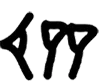into a Hindu-Arabic number.

1.
Convert the Babylonian number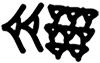into a Hindu-Arabic number.

### Who Knew?

#### The Legacy of Babylonian System

The Babylonian system can still be seen today. An hour is 60 minutes, and a minute is 60 seconds. Additionally, when measuring angles in degrees, each degree can be split into 60 minutes (1/60th of a degree) and 60 seconds (1/60th of a minute).

### Understand and Convert Mayan Numerals to Hindu-Arabic Numerals

The Mayans employed a positional system just as we do and the Babylonians did, but they based their position values on powers of 20 and they had a dedicated symbol for zero. Similar to the Babylonians, the Mayans would repeat symbols to indicate certain values. A single dot was a 1, two dots were a 2, up to four dots. Then a five was a horizontal bar. The horizontal bars could be used three times, since the fourth horizontal bar would make a 20, which was a new position in the number. The 0 was a special picture, which appears like a turtle lying on its back. The shell would then be "empty," so maybe that’s why the symbol was 0. The complete list is provided in Table 4.2. Another feature of Mayan numbers was that they were written vertically. The powers of 20 increased from bottom to top.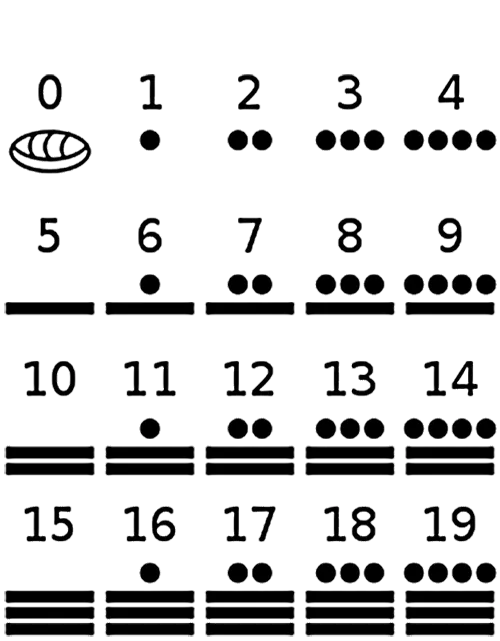Table 4.2 Mayan Numerals

To summarize, the Mayan system of numbers used repeating symbol to indicate more than one, used place values, and employed a 0. So how do we convert from Mayan numbers to Hindu-Arabic numbers? To do so, we need to use the symbols from Table 4.2 and then place values based on powers of 20. If you have $nn$ digits in the Mayan number, you multiply the first “digit” by 20 raised to one less than the number of “digits.” You then continue through the “digits,” multiplying each by 20 raised to a power that is one smaller than the previous power. Fortunately, there is an explicit 0, so there is no ambiguity about numbers like 110, 101, and 11.

### Example 4.7

#### Converting Two-Digit Mayan Numbers to Hindu-Arabic Numbers

Convert the Mayan number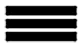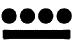into a Hindu-Arabic number.

1.
Convert the Mayan number into a Hindu-Arabic number.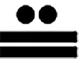### Example 4.8

#### Converting Three-Digit Mayan Numbers to Hindu-Arabic Numbers

Convert the Mayan number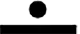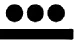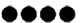into a Hindu-Arabic number.

1.
Convert the Mayan number into a Hindu-Arabic number.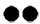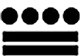### Example 4.9

#### Converting Four-Digit Mayan Numbers to Hindu-Arabic Numbers

Convert the Mayan number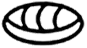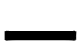into a Hindu-Arabic number.

1.
Convert the Mayan number into a Hindu-Arabic number.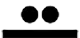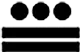### Who Knew?

#### The Mayan Calendar

The Mayans used this base 20 system for everyday situations. But their culturally important, and extremely accurate, calendar system used a slightly different system. For their calendars, they used a system where the place values were 1, 20, then 20*18, then 20*18*18. The reason for this is 20*18 is 360, which is closer to the number of days in a year. Had they used a purely base 20 system for their calendar, they’d be very far off with 400 days in a year.

Three hundred sixty days still left the Mayans a bit short, as there are 365 days in a year (ignoring leap years). The Mayan calendar also included 5 days, called Wayeb days, which brings their calendar to 365 days. As it happens, Wayeb is the Mayan god of misfortune, so these 5 days were considered the bad luck days.

### Understand and Convert Between Roman Numerals and Hindu-Arabic Numerals

The Mayan and Babylonian systems shared two features, one of which we are familiar with (place value) and one that we don’t use (repeated symbols). The Roman system of numbers used repeated symbols, but does not employ a place value. It also lacks a 0. The Roman system is built on the following symbols in Table 4.3.

Roman Numeral Hindu-Arabic Value
I 1
V 5
X 10
L 50
C 100
D 500
M 1,000
Table 4.3 Roman Numerals

As in the Mayan and Babylonian systems, a symbol may be repeated to indicate a larger value. However, at 4, they did not use IIII. They instead used IV. Since the I came before the V, the number stands for “one before five.” A similar process was used for 9, which was written IX, or “one before ten.” The value 40 was written XL, or “ten before fifty,” while 49 was written XLIX, or “forty plus nine.”

The following are the rules for writing and reading Roman numerals.

• The representations for bigger values precede those for smaller values.
• Up to three symbols may be grouped together; for example, III for 3, or XXX for 30, or CC for 200.
• A larger value followed by a smaller value indicated addition; for example, VII for 7, XIII for 13, LV for 55, and MCC for 1200.
• I can be placed before V to indicate 4, or before X, to indicate 9. These are the only ways I is used as a subtraction.
• X can be placed before L to indicate 40, and before C to indicate 90. These are the only ways X is used as a subtraction.
• C can be placed before D to indicate 400, and before M to indicate 900. These are the only ways C is used as a subtraction.
• If multiple symbols are used, and a subtraction involving that symbol, the subtraction part comes after the multiple symbols. For example, XXIX for 29 and CCXC for 290.

### Who Knew?

#### Legacy of Roman Numerals

The Roman numbering system is still used today in some situations. Many cornerstones of buildings have the year written in Roman numerals. Movie titles often represent the year the movie was produced as Roman numerals. The most recognizable might be that the Super Bowl is numbered using Roman numerals.

### Example 4.10

#### Converting Roman Numerals to Hindu-Arabic Numbers

Convert the following Roman numerals into Hindu-Arabic numerals.

1. XXVII
2. XXXIV
3. MMCMXLVIII

Convert the following Roman numerals into Hindu-Arabic numerals.
1.
LXXVII
2.
CCXL
3.
MMMCDXLVII

### Video

Of course, we can convert from Hindu-Arabic numerals, to Roman numerals, too.

### Example 4.11

#### Converting Hindu-Arabic Numbers to Roman Numerals

Convert the following Hindu-Arabic numerals into Roman numerals.

1. 38
2. 94
3. 846
4. 2,987

Convert the following Hindu-Arabic numerals into Roman numerals.
1.
27
2.
49
3.
739
4.
3,647

### Video

7.
What is the place value for Babylonian numerals?
8.
What place value is used in the Mayan numeration system?
9.
What place value is used for Roman numerals?
10.
Convert the Babylonian numeralinto a Hindu-Arabic numeral.
11.
Convert the Mayan numeral into a Hindu-Arabic numeral.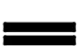12.
Convert the Roman numeral CCXLVII into a Hindu-Arabic numeral.
13.
Convert 479 into a Roman numeral.

### Section 4.2 Exercises

For the following exercises, convert the Babylonian numeral into a Hindu-Arabic numeral.
1 .2 .3 .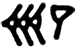4 .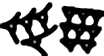5 .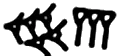6 .7 .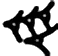8 .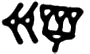For the following exercises, express the Mayan numeral as a Hindu-Arabic numeral. Use the common system, which is based on powers of 20 only.
9 .10 .11 .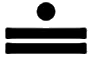12 .13 .14 .15 .16 .For the following exercises, express the Roman numeral as a Hindu-Arabic numeral.
17 .
VII
18 .
XI
19 .
IX
20 .
XXIV
21 .
MCXLII
22 .
CXXII
23 .
DCCXLIV
24 .
MCMLIX
For the following exercises, express the Hindu-Arabic numeral as a Roman numeral.
25 .
8
26 .
14
27 .
27
28 .
94
29 .
274
30 .
487
31 .
936
32 .
2,481
33 .
What uses a place value system for numbers: Roman, Babylonian, Egyptian, Greek?
34 .
What uses an additive system: Roman, Mayan, Egyptian, Greek?
35 .
What uses a 0: Roman, Mayan, Egyptian, Greek?
Order a print copy

As an Amazon Associate we earn from qualifying purchases.